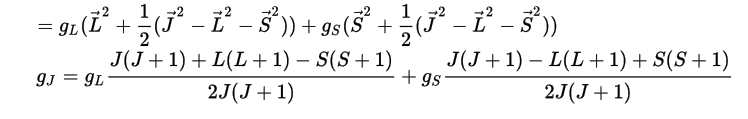# Basic derivation of g factorI was just going through the calculation to go from the top line to the bottom and was just not arriving at the same result. Working backwards and just looking at the first term (i.e. the one with coefficient ##g_L## I get):

## \frac {J^2 + J + L^2 + L - S^2 - S}{2(J^2 + J)} = \frac {L^2 + S^2 + 2LS + L + S + L^2 + L - S^2 - S}{2(L^2 + S^2 + 2LS + L + S)} = \frac{L^2 + LS + L}{J(J + 1)} ## (assuming L and S commute)

Although this is not equivalent to the above expression and it appears I am missing something very basic. Any help with this would be great.

Homework Helper
Gold Member
These angular momentum operators behave in a somewhat odd fashion, e.g. ## \vec{L}^2=(L+1)(L) (\hbar)^2 ## and ##\vec{S}^2=(S+1)(S)(\hbar)^2 ## and ## \vec{J}^2= (\vec{L}+\vec{S})^2=\vec{L}^2+2 \vec{L} \cdot \vec{S}+\vec{S}^2 ##. From this last expression, one can solve for ## \vec{L} \cdot \vec{S} ##. The ## g_J ## factor is found by computing the component of ## \vec{L} ## along ## \vec{J} ## by taking ## \vec{L} \cdot \vec{J}/|\vec{J}| ## and putting it in the ## \vec{J}/|\vec{J}| ## direction. The result is a ## |\vec{J}^2|=(J+1)(J)(\hbar)^2 ## in the denominator. (Similarly for the ## S ## term, with a ## g_L ## on the ## L ## term and a ## g_S ## on the ## S ## term.)

Last edited:
These angular momentum operators behave in a somewhat odd fashion, e.g. ## \vec{L}^2=(L+1)(L) (\hbar)^2 ## and ##\vec{S}^2=(S+1)(S)(\hbar)^2 ## and ## \vec{J}^2= (\vec{L}+\vec{S})^2=\vec{L}^2+2 \vec{L} \cdot \vec{S}+\vec{S}^2 ##. From this last expression, one can solve for ## \vec{L} \cdot \vec{S} ##. The ## g_J ## factor is found by computing the component of ## \vec{L} ## along ## \vec{J} ## by taking ## \vec{L} \cdot \vec{J}/|\vec{J}| ## and putting it in the ## \vec{J}/|\vec{J}| ## direction. The result is a ## |\vec{J}^2|=(J+1)(J)(\hbar)^2 ## in the denominator. (Similarly for the ## S ## term, with a ## g_L ## on the ## L ## term and a ## g_S ## on the ## S ## term.)

Thank you for the explanation. It appears in this link (towards the end, above the equations I posted above) that they mistakenly include the magnitude of ## \vec{J}##and did not only consider its direction.